Random Sampling using RANDOM Random Sampling using RANDOM

• Slides: 14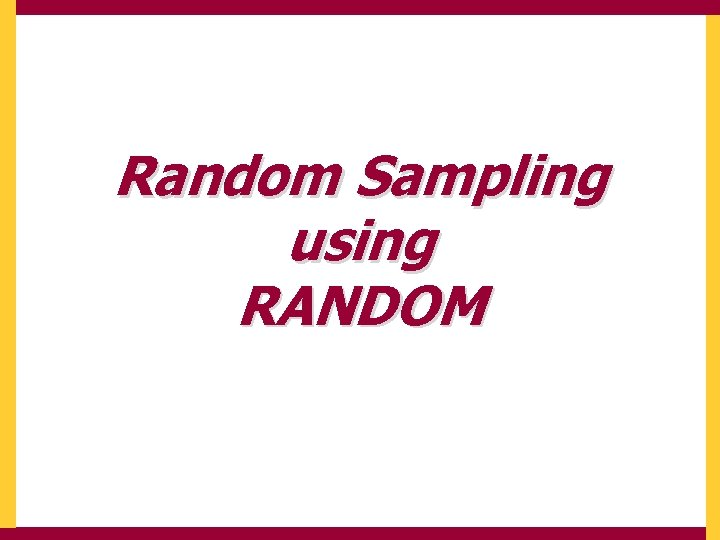Random Sampling using RANDOMRandom Sampling using RANDOM Random: Generates a pseudo random number to 3 decimal places that is less than 1. i. e. it generates a random number in the range [0, 1] RANDOM is in RedRandom Sampling using RANDOM We want to choose a RANDom number [0, 1] R – DICE [1, 6] R-COINS [1, 2] R-INT [0, 99]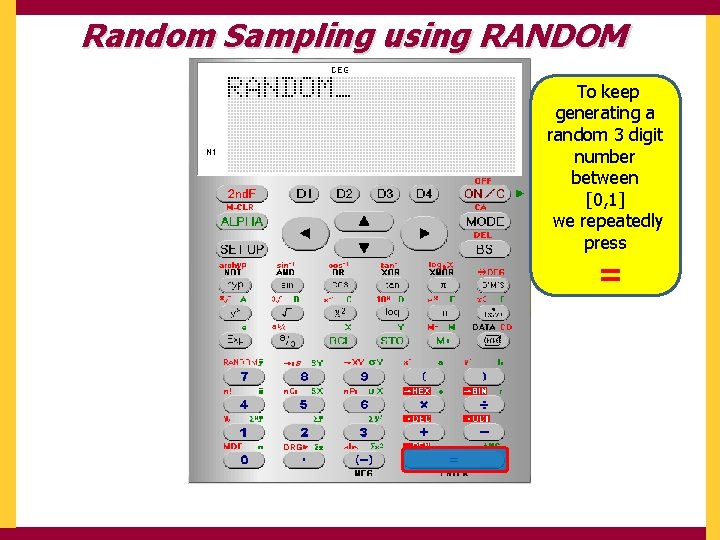Random Sampling using RANDOM To keep generating a random 3 digit number between [0, 1] we repeatedly press =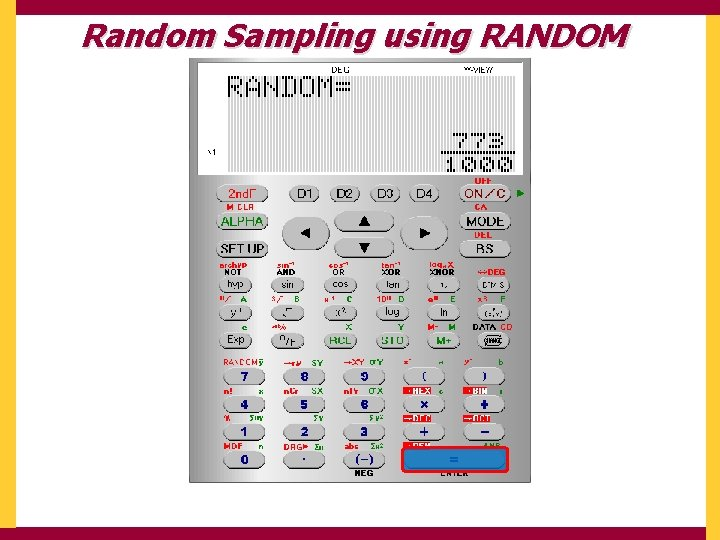Random Sampling using RANDOMRandom Sampling using RANDOM The calculator automatically displays in W View If we are generating lots of numbers this may become annoying. We need to SET UP the EDITOR into Linear Display (as a single line …Decimals)Random Sampling using RANDOM The calculator automatically displays in W View If we are generating lots of numbers this may become annoying. We need to SET UP the EDITOR into LINEar Display (as a single line …Decimals)Random Sampling using RANDOM We need to generate a RANDOM number again RANDOM is in Red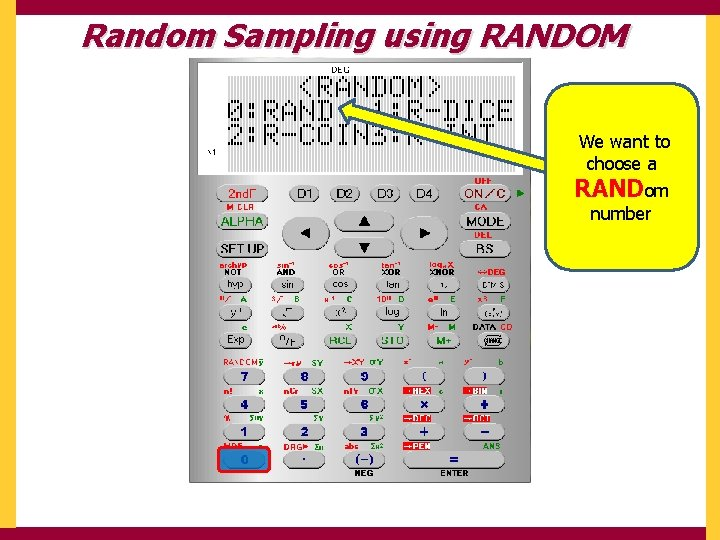Random Sampling using RANDOM We want to choose a RANDom numberRandom Sampling using RANDOM To keep generating a random 3 digit number between [0, 1] we repeatedly press =Random Sampling using RANDOMRandom Sampling using RANDOM for an interval [1, 200] If we multiply the randomly generated number by 199 then 199 x [0, 1] = [0, 199] To get it between 1 and 200 we must add 1 199 x [0, 1] + 1 = [1, 200] But we must first SET UP the calculator to Fix to 0 decimal placeRandom Sampling using RANDOM for an interval [1, 200] But we must first SET UP the calculator to Fix to 0 decimal place We want 0 decimal places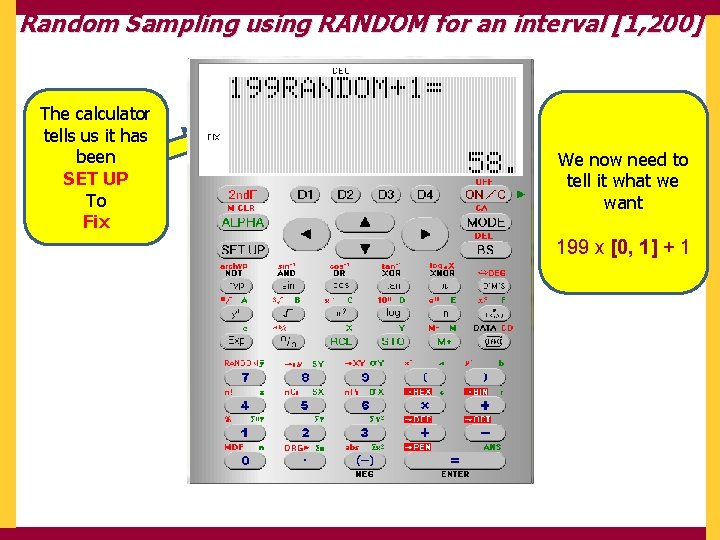Random Sampling using RANDOM for an interval [1, 200] The calculator tells us it has been SET UP To Fix We now need to tell it what we want 199 x [0, 1] + 1